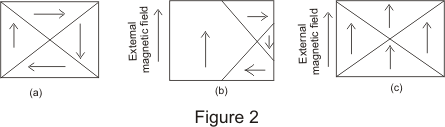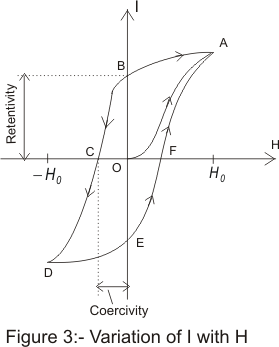Concept of ferromagnetism and hysteresis

(4) concept of ferromagnetism

• Ferromagnetism is the existence of the spontaneous magnetization ,even in the absence of an external magnetic field
• Internal magnetic field in ferromagnetism may be hundred or thousand times greater than that of diamagnetic and paramagnetic material
• Relation between I and H magnetization intensity and magnetic field is not linear. I and H are no longer have direct proportionality in case of ferromagnetic materials .hence magnetic susceptibility is very large but no longer constant
• Even in the absence of external field some ferromagnetic material exhibits large magnetization and can become permanent magnetized
• Some of the elements exhibiting ferromagnetic properties at room temperature are iron ,nickle,cobalt and gadolinium
• Because of complicated relation ship between I and H in case of ferromagnetic material, it is not possible to express I as a function of H
• So when a piece of unmagnetized iron is brought near a magnet or is subjected to the magnetic field of an electric current, the magnetization induced in iron by the field is described by a magnetization curve obtained by plotting the intensity of magnetization I against the field strength H
• Ferromagnetism can occur only in paramagnetic material i.e molecule and atoms of a ferromagnetic material also has unpaired electrons and hence non -zero permanent magnetic moment
• all ferromagnetic materials are composed of many small magnets or domains ,each of which consists of many atoms within a domain. Size of a domain is usually microscopic
• Within the domain, all magnetic moments are aligned ,but the alignment of magnetic moments varies from domain to domain which result in zero net magnetic moment of the macroscopic piece of material as a whole shown below in fig 2(a)• when the substance is placed in an external field ,magnetism of substance can increase in two different ways
i) By the displacement of the boundaries of the domain where domains oriented favorably with respect to the external field increase in and those oriented opposite to the external field are reduced in size as shown in fig 2(b)
ii) By the rotation of domain that is the domain rotate until their magnetic moments are aligned more or less in the direction of the externally applied magnetic field
• In presence of week magnetic field material is magnetized mostly by the displacement of the domains and in presence of strong fields magnetization takes place mostly by the rotation of the domains.
• In case of ferromagnetic materials on removal of the external magnetic field ,material is not completely demagnetized and some residual magnetization remains in it
• Every ferromagnetic material has a critical temperature known as curie temperature (Tc) above which material becomes paramagnetic and this transition of material from ferromagnetic to paramagnetic is a phase change or phase transition analogous to those between solid, liquid and gaseous phases of the matter

(5) Hysteresis

• We have already mentioned that in case of ferromagnetic materials ,the relation between I and H is not linear
• This relation can even depend on the history of the sample i.e whether it has been previously magnetized or not
• when we place a ferromagnetic material in the magnetic field it gets magnetized by induction
• If the field strength is first increased from a zero to high value and then decreased again, it is observed that the original curve is not retraced, the induction lags behind and follows a characteristics curve. This phenomenon is known as Hysteresis and characteristics curve is known as hysteresis loop as shown below in the curveFigure 3 shows the variation of I and H.In the beginning I= 0 and H=0 as represented by the point O in the figure. At this instant the sample is in unmagnetized state
• As value of H is increased, I also increases non uniformly .If we increase H indefinitely the intensity of magnetization of ferromagnetic material approaches finite limit known as saturation
• Thus at H=H0 ,the magnetization becomes nearly saturated and magnetization and magnetization varies along path OA
• Now if we begin to decrease the value of magnetic field ,the magnetization I of the substance also begin to decrease but this time not following the path AO but following a new path AB
• when H becomes equal to zero, I still have value equal to OB,This magnetization remaining in substance when magnetizing field becomes equal to zero is called the residual magnetism and the remaining value of I at point B is known as retentively of the material
• To reduce I to zero, we will increase field H in reverse direction and the magnetization I decreases following curve BC where at point C,I becomes equal to zero where H=OC
• The value OC of the magnetizing field is called coercivity of the substance
• the coercivity of the substance is a measure of the reverse magnetizing field required to bring magnetization I equal to zero
• if we further increase H beyond OC the sample begins to get magnetized in reverse direction ,again getting saturated at D at H=-H0
• while taking back H from its negative value through zero to its original maximum positive value H0,we symmetrical curve DEFA
• Thus we see that if the field strength is first increased from zero to saturated and then decreased again, it is observed that original curve is not retraced, the induction lags behind the field and follows a characteristic curve .This phenomenon is known as hysteresis and the characteristic curve ( Here ABCDEFA) is known as Hysteresis loop

Note to our visitors :-

Thanks for visiting our website.
DISCLOSURE: THIS PAGE MAY CONTAIN AFFILIATE LINKS, MEANING I GET A COMMISSION IF YOU DECIDE TO MAKE A PURCHASE THROUGH MY LINKS, AT NO COST TO YOU. PLEASE READ MY DISCLOSURE FOR MORE INFO.